# VLOOKUP Excel FunctionArticle byJeevan A Y## VLOOKUP Function in Excel

The VLOOKUP excel function looks for a specified value in a single column and then, it fetches and returns a corresponding value from the same row (as that of the specified value) of a different column. The value which is looked up is called the lookup value and the value that is returned is called a match (or the output). VLOOKUP stands for “vertical lookup” and this function is a part of the Lookup & Reference functions of Excel.

For example, in the following image, the following formula can fetch the salary of the “ID189” employee.

“=VLOOKUP(E2,\$A\$2:\$C\$11,3,0)”

The output is \$7,441. The VLOOKUP function looks for the value of cell E2 in the range A2:C11 of Excel. It returns an output from column C of row 8. This row is the same as that of the lookup value “ID189.”

###### Key Takeaways
• The VLOOKUP function of Excel can retrieve a value based on the supplied lookup value, table array, and column index number.
• The VLOOKUP function can perform a dynamic two-way lookup with the help of the MATCH function of Excel.
• The VLOOKUP function can search for a value from left to right in a column of Excel.

### VLOOKUP() Formula in Excel

The syntax of the VLOOKUP() function of Excel is given in the following image:

The VLOOKUP function accepts the following arguments:

• Lookup_value: This is the value to be searched. It can either be supplied directly or as a cell reference containing the “lookup_value.” If one is supplying a text value directly to the function, it must be enclosed within double quotation marks. The “lookup_value” must necessarily be in the first column of the “table_array.”
• Table_array: This is the range where the lookup value is to be searched. This range can consist of numbers, text values, logical values (true and false), and dates. The VLOOKUP function always searches in the left-most column of the “table_array.” This column is called the lookup column.
• Col_index_num: This is the column of the “table_array” from which the value is to be returned. As a result, this column is called the return column. The “col_index_num” for the first (or the left-most) column of the “table_array” is 1, for the second column is 2, for the third column is 3, and so on.
• Range_lookup: This indicates whether to look for a partial match or an exact match. It can take the following values:
• TRUE or 1–This looks for a partial or an approximate match. In a partial match, the output matches the lookup value partially. However, when the “range_lookup” is set at “true,” the VLOOKUP first looks for an exact match. If an exact match is not found, the VLOOKUP function looks for the largest (or the closest) value, which is smaller than the lookup value.
• FALSE or 0–This looks for an exact match. In an exact match, the output matches the lookup value exactly. If an exact match is not found, the VLOOKUP function returns the “#N/A” error.

The “lookup_value,” “table_array,” and “col_index_num” arguments are mandatory, while the “range_lookup” is optional. If the “range_lookup” argument is omitted, the VLOOKUP function assumes it to be “true.”

Note 1: The lookup column should always be placed to the left of the return column. If not, the VLOOKUP function returns the “#N/A” error in Excel.

Note 2: Before setting the “range_lookup” argument as “true,” one needs to sort the lookup column in ascending order.

### How to Use the VLOOKUP Function in Excel?

Let us consider some examples of the VLOOKUP function to understand its working in Excel.

There are two images titled “image 1” and “image 2.” The following information is given:

• “Image 1” shows the IDs, names, and prices of a few products of an organization.
• “Image 2” shows the highlighted price of product ID “P1972.”

Fetch the price of the product “P1972” in cell E2. Find an exact match and supply the lookup value directly to the VLOOKUP function of Excel.

Image 1

Image 2

The steps to fetch an exact match by using the VLOOKUP function in Excel are listed as follows:

1. Open the VLOOKUP function in cell E2. For this, type “=VLOOKUP” followed by the opening parenthesis.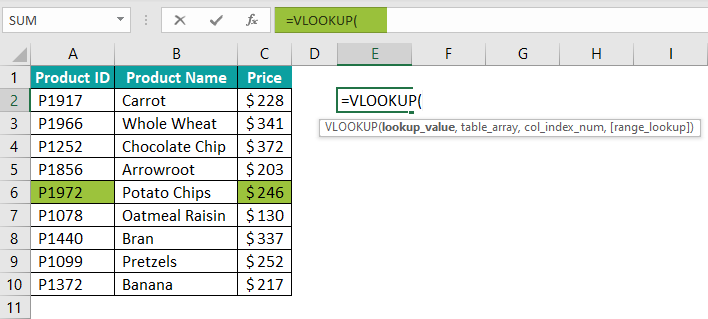2. Enter the product ID “P1972” in double quotation marks. The reason is that it is a text string and not a number. This product ID is the “lookup_value.”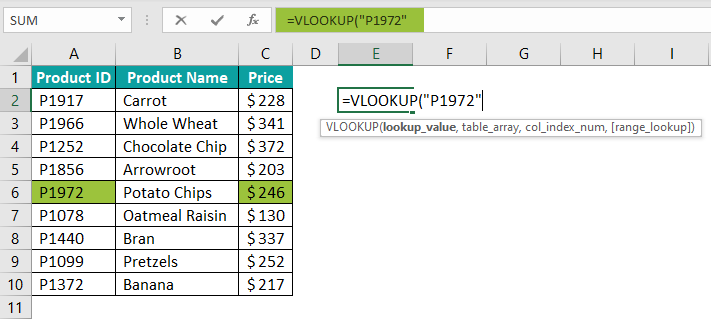3. Enter the range A2:C10. This is the “table_array” argument of the VLOOKUP function. We are trying to find the price of the product “P1972” in this range.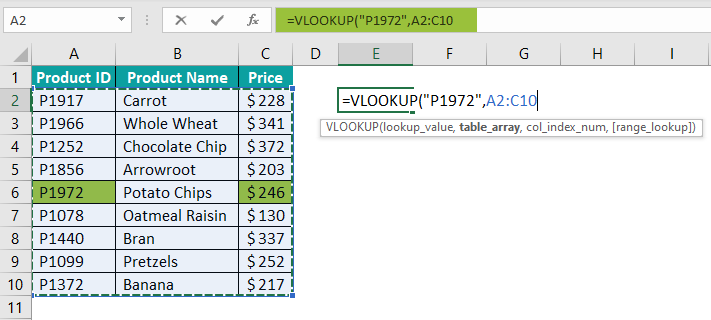4. Enter the “col_index_num” as 3. So, the output needs to be returned from the third column (column C) of the specified “table_array” (A2:C10).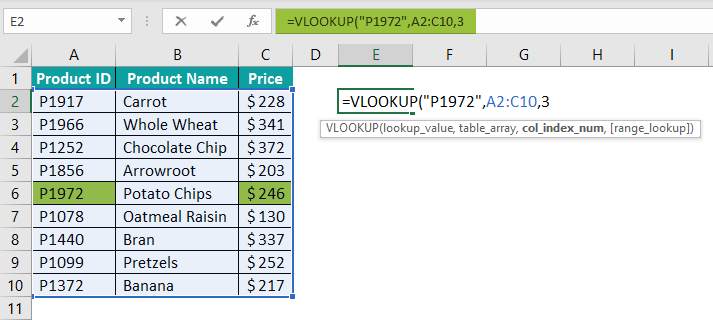5. Specify the “range_lookup” argument as “false” since we want to look for an exact match. Alternatively, one can enter it as 0.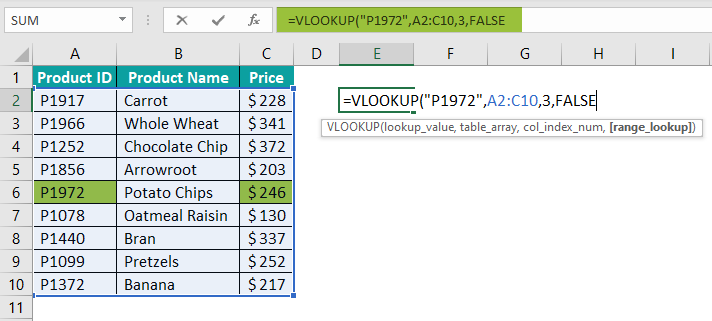6. Close the parenthesis and press the “Enter” key. The complete formula and the output are shown in the following image.

The VLOOKUP function looks for “P1972” in the range A2:C10 and returns an exact match from column C of this range. So, the price of the product “P1972” is \$246, which has been fetched in cell E2.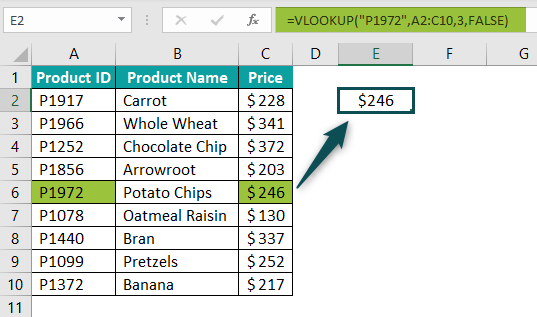### Vlookup Examples

#### Example #1–Supply the “Table_Array” as a Relative and Absolute Reference

Working on the dataset of “basic example,” we want to fetch the prices of products “P1252,” “P1440,” and “P1966” in cells F2, F3, and F4 respectively. Find an exact match with the VLOOKUP function of Excel.

Show the output by using the following references for the “table_array” argument:

• Relative cell references
• Absolute cell references

Explain the VLOOKUP formula used in the first bullet point.

Step 1: Enter the VLOOKUP formula in cell F2. For this, type “=VLOOKUP” followed by the opening parenthesis. Since the values to be looked up are placed in the range E2:E4, enter the first “lookup_value” as E2.

Step 2: Select the range A2:C10 for the “table_array” argument. Notice that this range has been entered as a relative reference.

Note: A relative reference changes when the formula is copied to the other cells, unlike an absolute reference that does not change on copying the formula.

Step 3: Enter the “col_index_num” as 3 because the price needs to be fetched from the third column (column C) of the “table_array” (A2:C10).

Step 4: Specify the “range_lookup” argument as “false.” This is because an exact match needs to be found. Next, press the “Enter” key.

The complete formula and the output (in cell F2) are shown in the following image.

Step 5: Drag the formula of cell F2 till cell F4 by using the fill handle. The outputs are shown in the following image.

Explanation of the formula: The VLOOKUP formula of cell F4 has returned the “#N/A” error even though the product “P1966” exists in the given dataset.

The reason behind this error is the usage of relative references in the “table_array” argument. This argument was entered as A2:C10 (in step 2), but since it is relative, it changed to A4:C12 on copying the formula to cell F4.

So, the range of the “table_array” kept moving downwards and by the time it reached the last product ID (P1966), the range became partially blank. Notice that the range A11:C12 of the following image is a blank range.

A solution for this error is to supply absolute references as the “table_array.”

Step 6: Select the range A2:C10 either in the formula bar or in the VLOOKUP formula of cell F2. Press the key F4 once and the dollar signs (\$) appear before the column and row coordinates. This implies that the references have become absolute.

Step 7: Drag the formula of cell F2 till cell F4. The outputs are shown in the following image. Hence, the correct prices have been fetched for the three product IDs.

Likewise, in case of multiple lookup values, we can use absolute references for the “table_array” argument. This will lock the references and give accurate results.

However, notice that the “lookup_value” has been entered as a relative reference. The relative reference allows the “lookup_value” to change as the VLOOKUP formula is copied downwards.

#### Example #2–Perform a Two-Way Lookup with the VLOOKUP and MATCH Functions

The following image shows the IDs, names, and revenues generated in five months by certain products. We want to perform the following tasks in Excel:

• Fetch the revenue generated by product “P1440” in March. The output should display in cell J2.
• Show what happens when the value in cell J1 is changed to “Apr” instead of “Mar.”
• Perform a two-way lookup to fetch the revenue of product “P1440” for April. The output should display in cell J2.
• Show what happens when the month in cell J1 is changed to “May.”

Use the VLOOKUP function of Excel for the first two bullet points. Use a combination of the VLOOKUP and MATCH excel functions for the last two bullet points. Explain the VLOOKUP and MATCH formula thus used.

Note: In a two-way lookup, the value at the intersection of a row and column needs to be fetched.

The steps to perform the given tasks are listed as follows:

Step 1: Open the VLOOKUP function in cell J2, as shown in the following image.

Step 2: Specify the “lookup_value” as cell reference I2. This is because “P1440” is in this cell.

Step 3: Enter the “table_array” argument by selecting the range A2:G10. Make this reference absolute by pressing the F4 key once.

Step 4: Specify the “col_index_num” as 5. This is because the value needs to be returned from the fifth column, which is “Mar” (or column E).

Step 5: Enter the “range_lookup” argument as “false” or 0. Next, close the parenthesis and press the “Enter” key. The output is \$320, as shown in the following image.

Hence, for the product “P1440,” the revenue of March has been correctly obtained.

Step 6: Change the month in cell J1 to “Apr.” Notice that cell J2 still shows the revenue of March. The revenue is not updated because the “col_index_num” was entered manually in the VLOOKUP function.

To enter a dynamic “col_index_num,” enter the MATCH function within the VLOOKUP function. By doing this, each time the month (in cell J1) changes, the “col_index_num” and the output also change.

Step 7: Type “MATCH” in place of the “col_index_num” argument of the VLOOKUP function.

Note: The MATCH function returns the relative position of a “lookup_value” within a “lookup_array.” The position of the first occurrence of the “lookup_value” is returned.

Step 8: Select cell J1 as the “lookup_value” of the MATCH function.

Step 9: Specify the range A1:G1 as the “lookup_array” of the MATCH function.

Step 10: Enter the “match_type” as 0, which means that an exact match is to be found. Close the parenthesis as all arguments of the MATCH function have been entered.

Step 11: Enter 0 as the “range_lookup” argument of the VLOOKUP function. This will instruct the VLOOKUP to look for an exact match. Next, close the parenthesis and press the “Enter” key.

The output is \$204. Hence, the VLOOKUP and MATCH functions have fetched the correct value from cell F8.

Explanation of the formula: The MATCH function looks for the value of cell J1 in the range A1:G1. It returns the output 6, which is the position of April in the stated range. This output becomes the “col_index_num” of the VLOOKUP function. The VLOOKUP looks for the value in cell I2 and returns a corresponding value from row 8 of column 6 (or cell F8).

Step 12: Change the month in cell J1 to May. Press the “Enter” key. Accordingly, the output is updated in cell J2. This time the revenue of May is displayed, which is \$420.

This is how a two-way lookup is performed with the VLOOKUP and MATCH functions of Excel.

#### Example #3–Supply the “Lookup_Value” by Removing the Trailing Spaces

Working on the dataset of example #2, we have retained only the sales of January in column C of the succeeding image. Further, a trailing space has been entered in cell E2. We want to perform the following tasks by using the VLOOKUP function of Excel:

• Show what happens when cell E2 (with the trailing space) is supplied as the “lookup_value.”
• Apply the TRIM function to fetch the correct revenue of product “P1440” in cell F2.

The steps to perform the given tasks are listed as follows:

Step 1: Enter the following formula in cell F2.

“=VLOOKUP(E2,\$A\$2:\$C\$10,3,0)”

Press the “Enter” key. The output is shown in cell F2. The “#N/A” error appears because the “lookup_value” of cell E2 (P1440) does not match the value of cell A8 exactly.

Step 2: The trailing space of cell E2 is shown in the following image. To see it, double-click within cell E2 or select this cell and press F2.

Step 3: To fetch the correct revenue of product “P1440,” enter the following formula in cell F2.

“=VLOOKUP(TRIM(E2),\$A\$2:\$C\$10,3,0)”

Press the “Enter” key. The output is \$306 in cell F2.

The TRIM function removes the unwanted spaces from cell E2. As a result, the correct sales of the stated product have been fetched in cell F2.

### Important Things to Note

The important points related to the VLOOKUP function of Excel are listed as follows:

• It searches for values only from left to right in a column.
• It returns the “#N/A” error if an exact match is not found in the dataset.
• It returns the “#REF” error if the supplied “col_index_num” is greater than the number of columns of the “table_array.”

### Frequently Asked Questions

1. Define the VLOOKUP function of Excel.

The VLOOKUP function of Excel searches for a value in a column and returns a corresponding match from the same row of another column.

For instance, in the following image, the VLOOKUP can fetch the units sold for the item “pen.”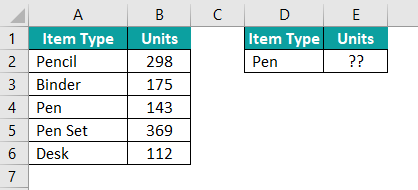The following formula is entered in cell E2.
=VLOOKUP(D2,\$A\$2:\$B\$6,2,0)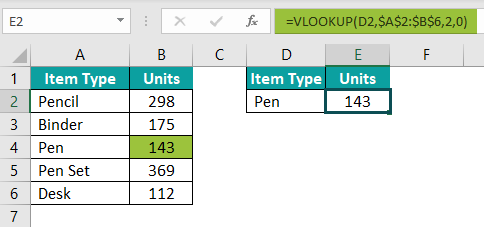The VLOOKUP looks for “pen” in the range A2:B6 and fetches an exact match, which is 143.

2. Why does the VLOOKUP function not work in Excel?

The reasons the VLOOKUP function may not work in Excel are listed as follows:
a. The return column may be to the right of the lookup column
b. The “col_index_num” provided may be outside the range of the “table_array”
c. The “lookup_value” may not match the value of the “table_array” exactly

3. Why is the VLOOKUP function used in Excel?

The VLOOKUP function of Excel helps find a corresponding match for the desired “lookup_value” of a “table_array.” To make VLOOKUP work, the data should be organized vertically in columns.